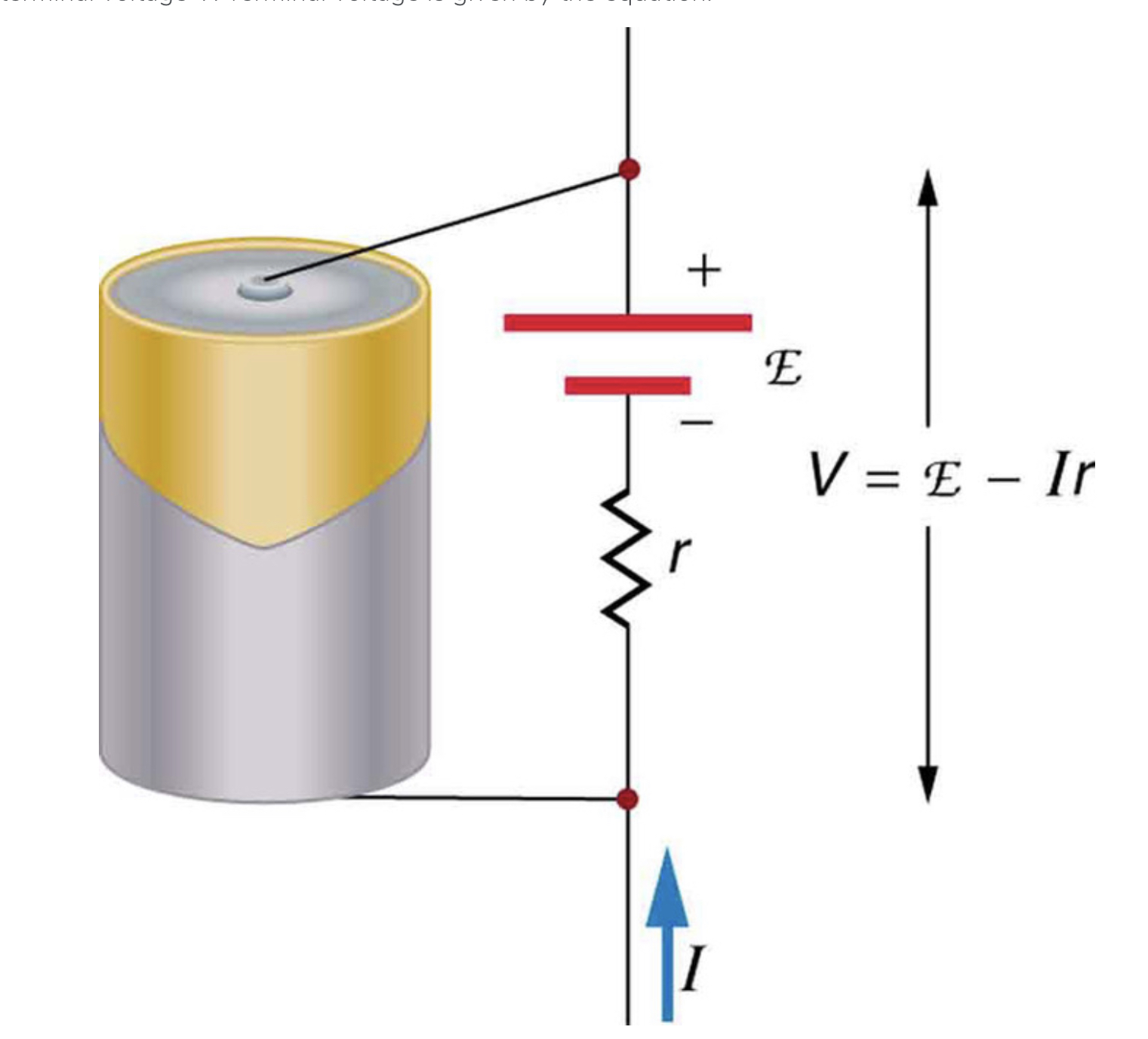Having trouble writing your personal statement?

MCAT Content / Circuit Elements / Electromotive Force Voltage

### Electromotive force, voltage

Topic: Circuit Elements

Electromotive force (emf) is the potential difference of a source when no current is flowing. Terminal voltage is the voltage output of a device is measured across its terminals.

Electric potential difference creates an electric field that exerts force on charges, causing current. We call this potential difference the electromotive force (emf). Emf is not a force at all; it is a special type of potential difference of a source when no current is flowing. Emf is directly related to the source of potential difference, such as the particular combination of chemicals in a battery. Units of emf are volts.All voltage sources create a potential difference and can supply current if connected to a resistance. However, emf differs from the voltage output of the device when current flows. The voltage across the terminals of a battery, for example, is less than the emf when the battery supplies current, and it declines further as the battery is depleted or loaded down. However, if the device’s output voltage can be measured without drawing current, then output voltage will equal emf (even for a very depleted battery).

The voltage output of a device is measured across its terminals and is called its terminal voltage V. Terminal voltage is given by the equation:

V = E – Ir,

– E stands for emf.
– r is the internal resistance
– I is the current flowing at the time of the measurement.

I is positive if current flows away from the positive terminal. The larger the current, the smaller the terminal voltage. Likewise, it is true that the larger the internal resistance, the smaller the terminal voltage.

Practice Questions

Electric Field Treatments and electroporation

MCAT Official Prep (AAMC)

Physics Online Flashcards Question 6

Practice Exam 1 C/P Section Passage 3 Question 14

Key Points

• Emf is the potential difference of a source when no current is flowing.

• Terminal voltage: the voltage output of a device is measured across its terminals V = E – Ir.

• Unit of emf and voltage is Volt( V)

Key Terms

Electric potential: amount of work needed to move a unit of charge from a reference point to a specific point inside the field without producing an acceleration

Electric field: a region around a charged particle or object within which a force would be exerted on other charged particles or objects.

Current: is the amount of charge moving through a cross section, over a period of time.

Voltage: electrical potential difference, expressed in Volts

Resistance: Resistance is a measure of the opposition to current flow in an electrical circuit.

Billing Information